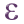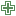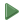# How can I calculate identifier TRAM field for this polylines

I would like to have any id field `TRAM`, together lines by group between 2 points, names (PE....). The idea is to write an expression using field calculator to get their id.

• The lines are always connected and touch at start/end points. The X,Y coords of the end point of one line is always equal to the X,Y coords of the next line's start point.
• The `fid` field is not necessarily sequential.
• The lines can have 0, 1 or 2 intersections with features on my point layer which are labeled PE(...).

Next step is use a dissolve algorithm to merge this `"TRAM"` group lines.

This is result I would like.EDIT 14 APRIL 2023

I have a point layer "PE", with different code, PE1,PE2,PE3 represent boxes. The line layer "OC", with different sections, always then are touching and intersects with point layer boxes.

I would like to add a field "TRAM" (I use field calculator) to create groups code for example (A,B,C... or other like "fid" 11,11,11,11,15,15,15,18,19) see image. I think that need x,y start and end point coordinates to know is a touch line.

How one image is better than my English words, here image:Here GeoPackage with both layers:

Here similar Question, but not work because I need X,Y start and end points of line for group code

• Question unclear. Have a look at aggregate tool. Apr 7 at 18:39
• If I understand your question, you are trying to split the line layer at every point (PE####), and then merge the lines grouped by this kind of sections generated between to points of the point layer. I guess "TRAM" means "tramo", that's what I call section, my question is: How this groups of lines "tramos" are numbered?
– Mayo
Apr 7 at 20:07
• And, in the picture it looks like there's a branching in the line layer near PE2903, if it's true, this will make it more complex.
– Mayo
Apr 7 at 20:51
• I'm also not very clear with what you're after, and the data in your screenshot has some line segments with duplicated `INI_POINT` coordinates... I get a feeling Aggregate tool or Virtual Layers would be a better tool for what you are after Apr 8 at 0:10
• I try to explain better. I digitalize lines "Obra_civil_KMZ" with different values (tipus_seccio=S1,S2...) and other fields. These lines represent different pipers and start or end or not intersect with a point layer call "PE". I need to create a field "TRAM" with common "id value" between these point layers, for in future apply a model with refactorys and dissolve them. Apr 8 at 18:45

If you just want to group lines that are between points of the PE#### point layer and there's no branching, use this approach:

1. Dissolve the line layer with the Dissolve tool. Use the Keep disjoint features separate option (available since version 3.26)
2. If your version is lower than 3.26 and there was no Keep disjoint features separate option then run the Multipart to singleparts tool on the Dissolved layer, else skip this step.
3. Open the Geometry by expression tool.
- Set as Input layer the Dissolved layer (if you did step 2 it'll be called Single parts)
- Set the Output geometry type parameter to Line
- Click thebutton of the Geometry expression parameter, then go to the Function editor tab and add a file with thebutton. Now in this new file paste this code, and click theSave and Load Functions button:
``````@qgsfunction(args=-1, group='Arrays', referenced_columns=[])
def array_zip(arrays, feature, parent):
return list(map(list, zip(*arrays)))
``````

this code defines one function that will be used by the following expression. Return to the Expression tab and paste the following expression, remember to change 'Point layer name/id' with your values, click ok and run:

``````collect_geometries(
with_variable(
name:='section',
value:=array_sort(
with_variable(
name:='line_vertices',
value:=array_foreach(
array:=geometries_to_array(nodes_to_points(\$geometry)),
expression:=array(x(@element), y(@element))
),
expression:=array_foreach(
array:=overlay_intersects(
layer:='Point layer name/id', -- replace here with your values
expression:=array(\$x, \$y)
),
expression:=array_find(
array:=@line_vertices,
value:=@element
)
)
)
),
expression:=with_variable(
name:='substrings',
value:=array_zip(
array_slice(@section, 0, -2),
array_slice(@section, 1, -1)
),
expression:=array_foreach(
array:=@substrings,
expression:=make_line(
array_slice(
geometries_to_array(nodes_to_points(\$geometry)),
@element,
@element
)
)
)
)
)
)
``````

Finally, but it looks that the lines are still merged, this happens because it's a multiline geometry. So run the Multipart to singleparts algorithm in this layer (Modified geometries) and you'll be done.

Dissolve all lines, then split them at the points. You can do it in 3 simple steps:

1. Dissolve the layer

2. At each point, create a small auxiliary line, perpendicular to the initial line. Use Geometry by expression on the point layer with this expression:

`````` extend(
make_line (
\$geometry,
project (
\$geometry,
1,  -- use a small value
3. Use Split with lines: use the dissolved layer for `Input layer` and the auxiliary lines from step 2 as `Split layer`. Now create a new field with the Field calculator expression `\$id`.
Small, auxiliary black lines at each point, used to split the dissolved line: